# Basic Ploting with matplotlib¶

matplotlib is the most popular Python library for producing plots and other 2D data visualizations. It was originally created by John D. Hunter (JDH) and is now maintained by a large team of developers. It is well-suited for creating plots suitable for publication. It integrates well with IPython, thus providing a comfortable interactive environment for plotting and exploring data. The plots are also interactive; you can zoom in on a section of the plot and pan around the plot using the toolbar in the plot window.

## Display the probability density function (pdf)¶

In :
import numpy as np
from scipy.stats import norm
import matplotlib.pyplot as plt

r = norm.rvs(loc=0, scale=1, size=1000)

x = np.linspace(norm.ppf(0.01), #ppf stands for percentiles.
norm.ppf(0.99), 100)

fig, ax = plt.subplots(1, 1)
ax.plot(x, norm.pdf(x),
'blue', lw=5, alpha=0.6, label='norm pdf')
plt.show()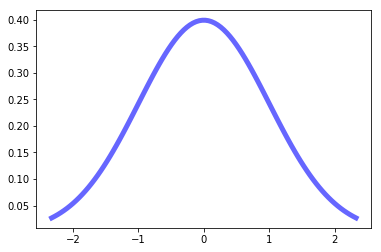And compare the histogram:

In :
fig, ax = plt.subplots(1, 1)
ax.hist(r, normed=True, histtype='stepfilled', alpha=1, label='...')
ax.legend(loc='best', frameon=False)
plt.show()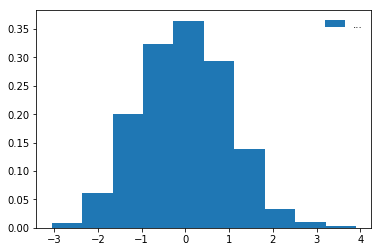In :
from matplotlib import pyplot as plt
years = [1950, 1960, 1970, 1980, 1990, 2000, 2010]
gdp = [300.2, 543.3, 1075.9, 2862.5, 5979.6, 10289.7, 14958.3]
# create a line chart, years on x-axis, gdp on y-axis
fig = plt.figure()
plt.plot(years, gdp, color='green', marker='o', linestyle='solid')
# add a title
plt.title("Nominal GDP")
# add a label to the y-axis
plt.ylabel("Billions of $") plt.show()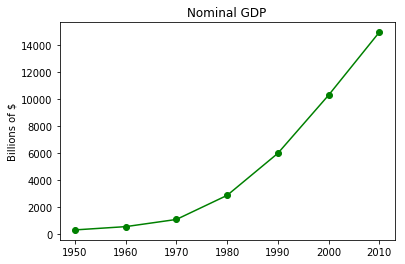## 3D Plot¶ In : from scipy import special def drumhead_height(n, k, distance, angle, t): kth_zero = special.jn_zeros(n, k)[-1] return np.cos(t) * np.cos(n*angle) * special.jn(n, distance*kth_zero) theta = np.r_[0:2*np.pi:50j] radius = np.r_[0:1:50j] x = np.array([r * np.cos(theta) for r in radius]) y = np.array([r * np.sin(theta) for r in radius]) z = np.array([drumhead_height(1, 1, r, theta, 0.5) for r in radius])  In : import matplotlib.pyplot as plt from mpl_toolkits.mplot3d import Axes3D from matplotlib import cm fig = plt.figure() ax = Axes3D(fig) ax.plot_surface(x, y, z, rstride=1, cstride=1, cmap=cm.jet) ax.set_xlabel('X') ax.set_ylabel('Y') ax.set_zlabel('Z') plt.show()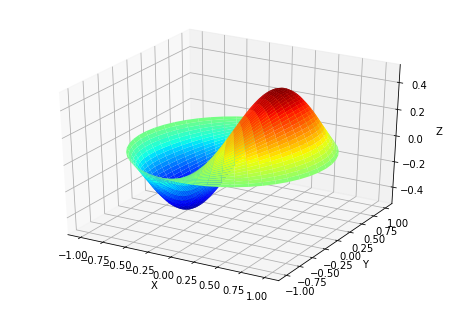# Statistical Data Visualization with Seaborn¶ Seaborn is a library for making attractive and informative statistical graphics in Python. It is built on top of matplotlib and tightly integrated with the PyData stack, including support for numpy and pandas data structures and statistical routines from scipy and statsmodels. Some of the features that seaborn offers are • Several built-in themes for styling matplotlib graphics • Tools for choosing color palettes to make beautiful plots that reveal patterns in your data • Functions for visualizing univariate and bivariate distributions or for comparing them between subsets of data • Tools that fit and visualize linear regression models for different kinds of independent and dependent variables • Functions that visualize matrices of data and use clustering algorithms to discover structure in those matrices • A function to plot statistical timeseries data with flexible estimation and representation of uncertainty around the estimate • High-level abstractions for structuring grids of plots that let you easily build complex visualizations ## Comparison with matplotlib¶ Seaborn should be thought of as a complement to matplotlib, not a replacement for it. When using seaborn, it is likely that you will often invoke matplotlib functions directly to draw simpler plots already available through the pyplot namespace. Further, the seaborn functions aim to make plots that are reasonably “production ready” (including extracting semantic information from Pandas objects to add informative labels), but full customization will require changing attributes on the matplotlib objects directly. The combination of seaborn’s high-level interface and matplotlib’s customizability and wide range of backends makes it easy to generate publication-quality figures. ## Visualizing the distribution of a dataset¶ When dealing with a set of data, often the first thing you’ll want to do is get a sense for how the variables are distributed. This chapter of the tutorial will give a brief introduction to some of the tools in seaborn for examining univariate and bivariate distributions. You may also want to look at the categorical plots chapter for examples of functions that make it easy to compare the distribution of a variable across levels of other variables. In : import numpy as np import pandas as pd from scipy import stats, integrate import matplotlib.pyplot as plt  In : import seaborn as sns sns.set(color_codes=True) np.random.seed(sum(map(ord, "distributions")))  ### Histograms¶ Histograms are likely familiar, and a hist function already exists in matplotlib. A histogram represents the distribution of data by forming bins along the range of the data and then drawing bars to show the number of observations that fall in each bin. To illustrate this, let’s remove the density curve and add a rug plot, which draws a small vertical tick at each observation. You can make the rug plot itself with the rugplot() function, but it is also available in distplot(): In : x = np.random.normal(size=100) sns.distplot(x);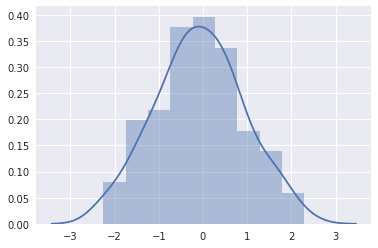In : sns.distplot(x, kde=False, rug=True);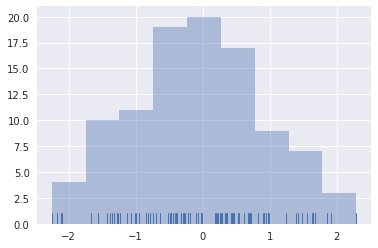In : import seaborn as sns import matplotlib.pyplot as plt sns.set(style="darkgrid") iris = sns.load_dataset("iris") # Subset the iris dataset by species setosa = iris.query("species == 'setosa'") virginica = iris.query("species == 'virginica'") # Set up the figure f, ax = plt.subplots(figsize=(8, 8)) ax.set_aspect("equal") # Draw the two density plots ax = sns.kdeplot(setosa.sepal_width, setosa.sepal_length, cmap="Reds", shade=True, shade_lowest=False) ax = sns.kdeplot(virginica.sepal_width, virginica.sepal_length, cmap="Blues", shade=True, shade_lowest=False) # Add labels to the plot red = sns.color_palette("Reds")[-2] blue = sns.color_palette("Blues")[-2] ax.text(2.5, 8.2, "virginica", size=16, color=blue) ax.text(3.8, 4.5, "setosa", size=16, color=red)  Out: Text(3.8,4.5,'setosa')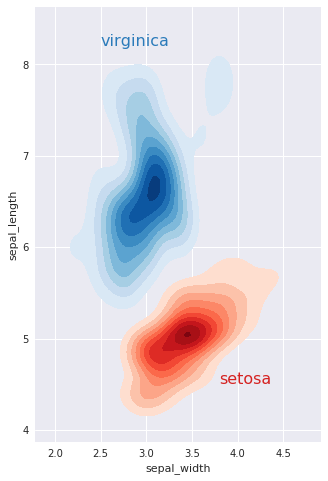### Kernel density estimation¶ It is also posible to use the kernel density estimation procedure to visualize a bivariate distribution. In seaborn, this kind of plot is shown with a contour plot and is available as a style in jointplot(): In : mean, cov = [0, 1], [(1, .5), (.5, 1)] data = np.random.multivariate_normal(mean, cov, 200) df = pd.DataFrame(data, columns=["x", "y"])  In : sns.jointplot(x="x", y="y", data=df, kind="kde");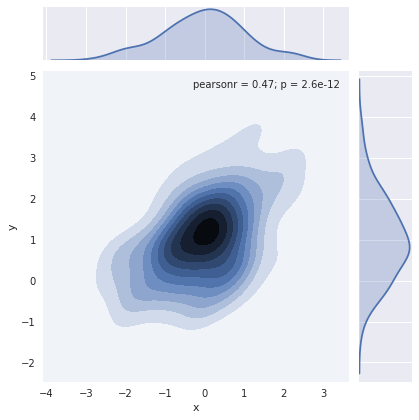You can also draw a two-dimensional kernel density plot with the kdeplot() function. This allows you to draw this kind of plot onto a specific (and possibly already existing) matplotlib axes, whereas the jointplot() function manages its own figure: In : f, ax = plt.subplots(figsize=(6, 6)) sns.kdeplot(df.x, df.y, ax=ax) sns.rugplot(df.x, color="g", ax=ax) sns.rugplot(df.y, vertical=True, ax=ax);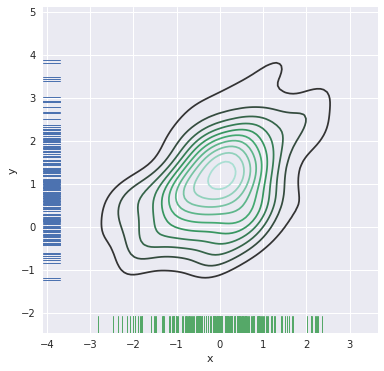The jointplot() function uses a JointGrid to manage the figure. For more flexibility, you may want to draw your figure by using JointGrid directly. jointplot() returns the JointGrid object after plotting, which you can use to add more layers or to tweak other aspects of the visualization: In : g = sns.jointplot(x="x", y="y", data=df, kind="kde", color="m") g.plot_joint(plt.scatter, c="w", s=30, linewidth=1, marker="+") g.ax_joint.collections.set_alpha(0) g.set_axis_labels("$X$", "$Y\$");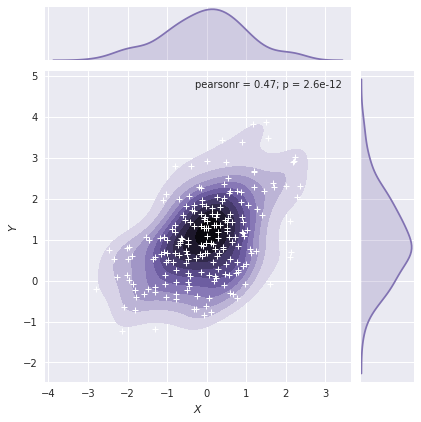### Visualizing pairwise relationships in a dataset¶

To plot multiple pairwise bivariate distributions in a dataset, you can use the pairplot() function. This creates a matrix of axes and shows the relationship for each pair of columns in a DataFrame. by default, it also draws the univariate distribution of each variable on the diagonal Axes:

In :
iris = sns.load_dataset("iris")
sns.pairplot(iris);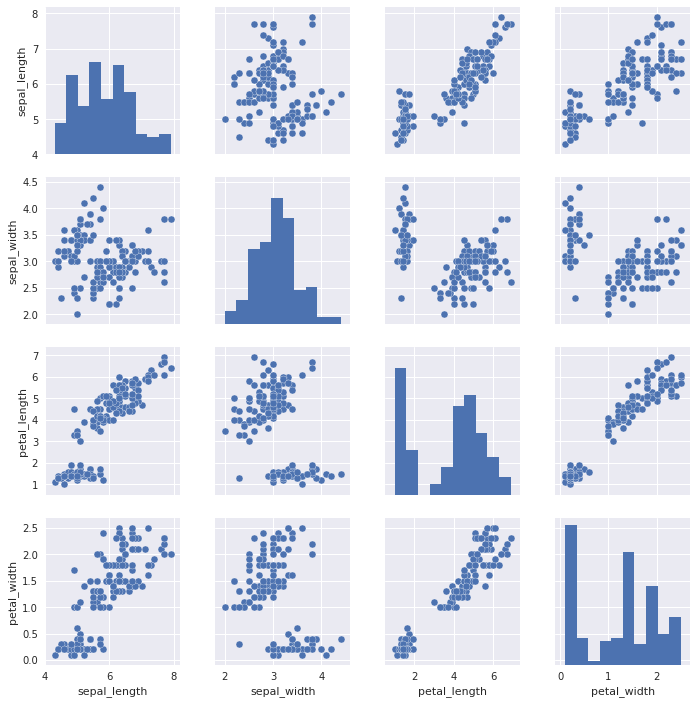Much like the relationship between jointplot() and JointGrid, the pairplot() function is built on top of a PairGrid object, which can be used directly for more flexibility:

In :
g = sns.PairGrid(iris)
g.map_diag(sns.kdeplot)
g.map_offdiag(sns.kdeplot, cmap="Blues_d", n_levels=6);

No handles with labels found to put in legend.
No handles with labels found to put in legend.
No handles with labels found to put in legend.
No handles with labels found to put in legend.
/usr/lib/python3/dist-packages/matplotlib/contour.py:967: UserWarning: The following kwargs were not used by contour: 'label', 'color'
s)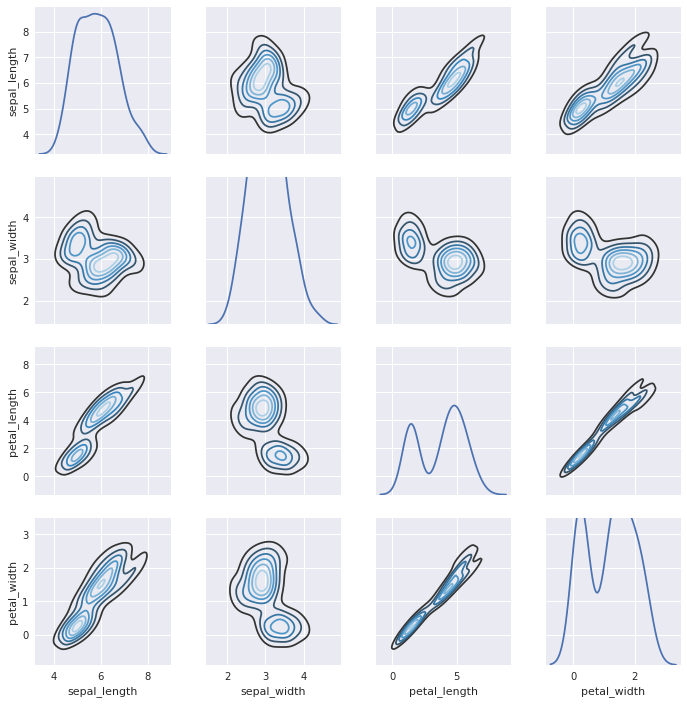PairGrid is flexible, but to take a quick look at a dataset, it can be easier to use pairplot(). This function uses scatterplots and histograms by default, although a few other kinds will be added (currently, you can also plot regression plots on the off-diagonals and KDEs on the diagonal).

You can also control the aesthetics of the plot with keyword arguments, and it returns the PairGrid instance for further tweaking.

In :
g = sns.pairplot(iris, hue="species", palette="Set2", diag_kind="kde", size=2.5)